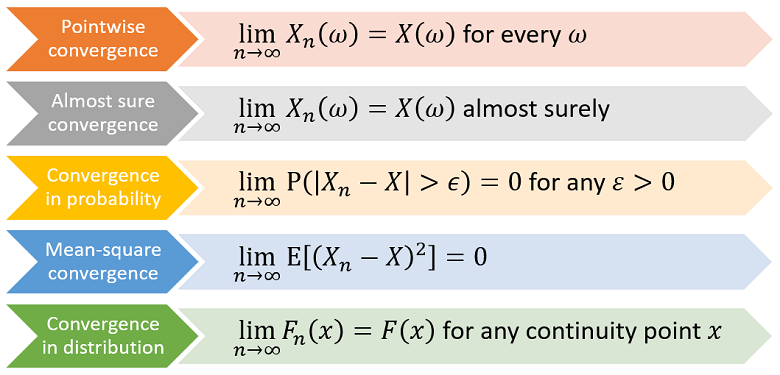StatLect

# Asymptotic theory

Learn the basics of asymptotic theory: how sequences of random variables and random vectors are characterized, how their convergence is defined, what conditions guarantee their convergence.

## Stochastic sequences

Generalizes the concepts introduced in the lecture on sequences of random variables

Various definitions, including IID, stationary, ergodic, mixing

## Modes of stochastic convergence

Convergence of almost all the sequences of real numbers obtained by fixing a sample point

Convergence of all the sequences of real numbers obtained by fixing a sample point

Convergence to zero of the mean-square distance between the limit and the terms of the sequence

Convergence to zero of the probability of being far from the limit

Relations among different concepts of stochastic convergence

Convergence of the distribution functions of the terms of the sequence to the distribution function of the limit## Theorems on stochastic convergence

Conditions guaranteeing that the sample average converges to a normal distribution

Conditions guaranteeing that the sample average converges to the true mean

An important application of the Continuous Mapping theorem

Stochastic convergence is preserved by continuous transformations

A method used to derive the asymptotic distribution of a function of an asymptotically normal sequence

## Empirical distributions

How to use the empirical distribution to approximate features of the original distribution

The distribution function of the discrete distribution of a sample of observations

A method used to reduce the variance of Monte Carlo estimates

Application of the plug-in principle to computer-generated samples

The books

Most of the learning materials found on this website are now available in a traditional textbook format.

Glossary entries
Share
• To enhance your privacy,
• we removed the social buttons,
• but don't forget to share.